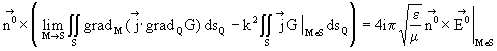Electro
Dynamics of
Elements of
Metall

# a program for electromagnetic fields calculating and researching into the electrodynamic properties of conducting structures

 The base of EDEM is formed by algorithms which were obtained as the result of development of the principles suggested by the authors for solving the problems under consideration.  The detailed description of this technique one may be found in [1,2].     In the current version of the program algorithms for solving the six types of problems are realized. These are plane two-dimensional problems for two kinds of polarization, a problems for surfaces of revolution with axisymmetric excitation; three-dimensional problems for structures of arbitrary shape, and also a problems for surfaces of revolution in which the incident field can be presented by quickly converging Fourier series by azimuthal coordinate.     The original problem is formulated as rigorous integral equations of first kind for surface current density induced on the analyzed structure by incident electromagnetic field. In case of three-dimensional problem the integral equation isTasks of other five classes are reduced to the one-dimensional integral equations or systems of such equations which have been written by a contour of cross-section of analyzed surfaces.    Kernels of the equations by the special representation for scalar potential are transformed to a form allowing subsequently greatly to reduce quantity of calculations. As a result of the numerical solution of the equations there are components of the currents induced on researched structure. Then various characteristics of the electromagnetic fields resulting from these currents are calculated    For the numerical solution it can be used both constant approximation, and the basic functions of higher order known as RWG functions.    For the solving of systems of linear algebraic equations both direct and iterative methods are realized, in particular the Generalized Minimal Residual Algorithm (GMREZ).    Besides the solving of the rigorous integrated equations, an approximate solving of tasks on the basis of a method of physical optics is possible. The sizes of researched structures can be at this very large. Doklady Akademii Nauk SSSR, 1984, v.276, N 1, p.96-100. Journal of Communications Technology and Electronics, Vol.45, Suppl.2, 2000, pp.S247-S259.
 Contacts:  info@edem3d.ruSite design and support: Defi